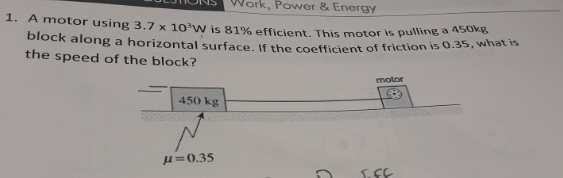# IONS Work, Power& Energy1. A motor using 3.7 x 10'W is 81% efficient. This motor is pulliblock along a horizontal surface. If the coefficient of tthe speed of the block?friction is 0.3s, what ismolor450o kg-0.35

Questionhelp_outlineImage TranscriptioncloseIONS Work, Power& Energy 1. A motor using 3.7 x 10'W is 81% efficient. This motor is pulli block along a horizontal surface. If the coefficient of t the speed of the block? friction is 0.3s, what is molor 450o kg -0.35 fullscreen
check_circleExpert Solution
Step 1

Given: work done = 3.7 × 103 W

Efficiency = 81%

mass of block, m = 450 kg

Coefficient of friction = 0.35

Find: Speed of the block

Step 2

To calculate speed of the block, use the relation as:

Power = Force × velocity

Step 3

Where, Power and Force can be ca...

### Want to see the full answer?

See Solution

#### Want to see this answer and more?

Solutions are written by subject experts who are available 24/7. Questions are typically answered within 1 hour*

See Solution
*Response times may vary by subject and question
Tagged in

### Work,Power and Energy